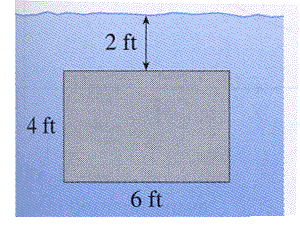A vertical plate is submerged in water and has the shape as seen in the picture. Using the fact that the weight of water is $62.5\; lbs/ft^3,$ calculate the hydrostatic force (in lbs) against the end of the tank.

(Hint: First set up a Riemann sum that approximates the hydrostatic force, which can then be used to obtain an integral that represents the force.)Hydrostatic force = lbs